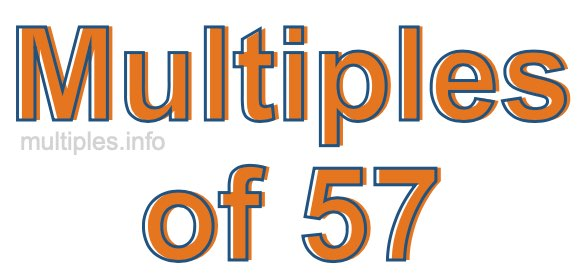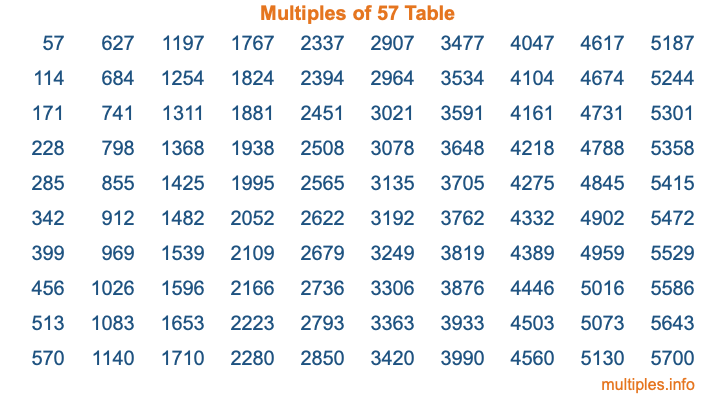Multiples of 57Welcome to the Multiples of 57 page. Here we will first teach you everything you will ever need to know about the multiples of 57, and then give you a study guide summary of everything we taught you to make sure you remember it all. Use this page to look up facts and learn information about the multiples of 57. This page will make you a multiples of fifty-seven expert!

Definition of Multiples of 57
Multiples of 57 are all the numbers that when divided by 57 equal an integer. Each of the multiples of 57 are called a multiple. A multiple of 57 is created by multiplying 57 by an integer.

Therefore, to create a list of multiples of 57, you start with 1 multiplied by 57, then 2 multiplied by 57, then 3 multiplied by 57, and so on for as long as you want. Thus, the list of the first five multiples of 57 is 57, 114, 171, 228, and 285. To see a larger list of multiples of 57, see the printable image of Multiples of 57 further down on this page. We also have a category where you can choose any nth multiple of 57.

Multiples of 57 Checker
The Multiples of 57 Checker below checks to see if any number of your choice is a multiple of 57. In other words, it checks to see if there is any number (integer) that when multiplied by 57 will equal your number. To do that, we divide your number by 57. If the the quotient is an integer, then your number is a multiple of 57.

Is  a multiple of 57?

Least Common Multiple of 57 and ...
A Least Common Multiple (LCM) is the lowest multiple that two or more numbers have in common. This is also called the smallest common multiple or lowest common multiple and is useful to know when you are adding our subtracting fractions. Enter one or more numbers below (57 is already entered) to find the LCM.

Check out our LCM Calculator if you need more details about the Least Common Multiple or if you need the LCM for different numbers for adding and subtraction fractions.

nth Multiple of 57
As we stated above, 57 is the first multiple of 57, 114 is the second multiple of 57, 171 is the third multiple of 57, and so on. Enter a number below to find the nth multiple of 57.

th multiple of 57

Multiples of 57 vs Factors of 57
57 is a multiple of 57 and a factor of 57, but that is where the similarities end. All postive multiples of 57 are 57 or greater than 57. All positive factors of 57 are 57 or less than 57.

Below is the beginning list of multiples of 57 and the factors of 57 so you can compare:

Multiples of 57: 57, 114, 171, 228, 285, etc.

Factors of 57: 1, 3, 19, 57

As you can see, the multiples of 57 are all the numbers that you can divide by 57 to get a whole number. The factors of 57, on the other hand, are all the whole numbers that you can multiply by another whole number to get 57.

It's also interesting to note that if a number (x) is a factor of 57, then 57 will also be a multiple of that number (x).

Multiples of 57 vs Divisors of 57
The divisors of 57 are all the integers that 57 can be divided by evenly. Below is a list of the divisors of 57.

Divisors of 57: 1, 3, 19, 57

The interesting thing to note here is that if you take any multiple of 57 and divide it by a divisor of 57, you will see that the quotient is an integer.

Multiples of 57 Table
Below is an image of the first 100 multiples of 57 in a table. The table is in chronological order, column by column. The first column has the first ten multiples of 57, the second column has the next ten multiples of 57, and so on.The Multiples of 57 Table is also referred to as the 57 Times Table or Times Table of 57. You are welcome to print out our table for your studies.

Negative Multiples of 57
Although not often discussed or needed in math, it is worth mentioning that you can make a list of negative multiples of 57 by multiplying 57 by -1, then by -2, then by -3, and so on, to get the following list of negative multiples of 57:

-57, -114, -171, -228, -285, etc.

Multiples of 57 Summary
Below is a summary of important Multiples of 57 facts that we have discussed on this page. To retain the knowledge on this page, we recommend that you read through the summary and explain to yourself or a study partner why they hold true.

There are an infinite number of multiples of 57.

A multiple of 57 divided by 57 will equal a whole number.

57 divided by a factor of 57 equals a divisor of 57.

The nth multiple of 57 is n times 57.

The largest factor of 57 is equal to the first positive multiple of 57.

57 is a multiple of every factor of 57.

57 is a multiple of 57.

A multiple of 57 divided by a divisor of 57 equals an integer.

57 divided by a divisor of 57 equals a factor of 57.

Any integer times 57 will equal a multiple of 57.

Multiples of a Number
Here you can get the multiples of another number, all with the same attention to detail as we did for multiples of 57 on this page.

Multiples of
Multiples of 58
Did you find our page about multiples of fifty-seven educational? Do you want more knowledge? Check out the multiples of the next number on our list!